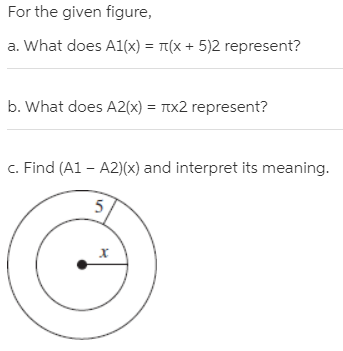# For the given figure, a. What does A1(x) = Tt(X + 5)2 represent? b. What does A2(x) = Ttx2 represent? c. Find (A1 – A2)(x) and interpret its meaning. 5,

Questionhelp_outlineImage TranscriptioncloseFor the given figure, a. What does A1(x) = Tt(X + 5)2 represent? b. What does A2(x) = Ttx2 represent? c. Find (A1 – A2)(x) and interpret its meaning. 5, fullscreen